Courses

# Fill Ups of Trigonometric Functions & Equations, Past year Questions JEE Advance, Class 11, Maths JEE Notes | EduRev

## JEE : Fill Ups of Trigonometric Functions & Equations, Past year Questions JEE Advance, Class 11, Maths JEE Notes | EduRev

The document Fill Ups of Trigonometric Functions & Equations, Past year Questions JEE Advance, Class 11, Maths JEE Notes | EduRev is a part of the JEE Course Maths 35 Years JEE Main & Advanced Past year Papers.
All you need of JEE at this link: JEE

Q.1. Suppose sin3x.sin 3x =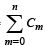cos mx is an identity in x, where C0, C1, ___Cn are constants, and Cn ≠ 0. Then the value of n is _________. (1981 - 2 Marks)
Ans. 6
Sol. sin3x . sin 3x =cos mx

sin3x.sin 3x =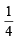[3 sinx – sin3x] sin 3x

=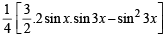=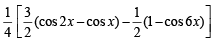=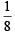[ cos 6x + 3 cos 2x - 3 cosx - 1]
We observe that on LHS 6 is the max value of m.
∴n = 6

Q.2. The solution set of the system of equations x + y =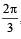, cosx + cosy =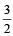where x and y are real, is _________. (1987 - 2 Marks)
Ans. φ
Sol. The equations are x + y = 2π /3 ... (i)

cos x + cos y = 3/2 ...(ii)

From eq.
(ii)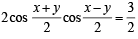⇒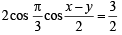[Using eq. (i)]

⇒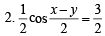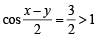Which has no solution.
∴ The solution of given equations is φ.

Q.3. The set of all x in the interval [0,π] for which 2sin2 x – 3 sin x + 1 ≥ 0, is _________. (1987 - 2 Marks)
Ans.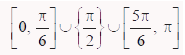Sol. We have  2 sin2 x – 3 sin x + 1 ≥ 0

⇒ (2 sin  x – 1)  (sin x – 1) ≥ 0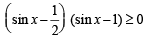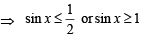But we know that sin x ≤ 1 and sin x ≥  0 for x ∈ [0, π]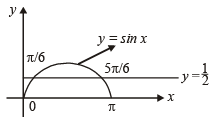⇒ either sin x = 1  or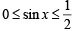⇒ either  x = π/2 or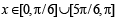Combining, we get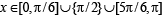Q.4. The sides of a triangle inscribed in a given circle subtend angles α, β and γ at the centre. The minimum value of the arithmetic mean of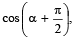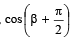and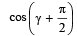is equal to _________. (1987 - 2 Marks)
Ans.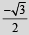Sol. We know that A.M. ≥ G.M.
⇒ Min value of AM. is obtained when AM = GM
⇒ The quantities whose AM is being taken are equal.

i.e., Cos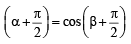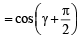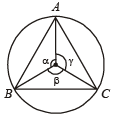⇒ sin α = sin β = sinγ

Also α +β + γ= 360° ⇒ α = β = γ=120° = 2π/3

∴ Min value of A.M.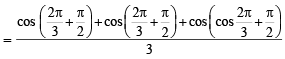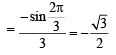Q.5. The value of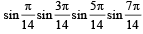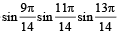is equal to _________. (1991 - 2 Marks)
Ans.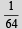Sol.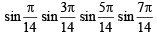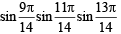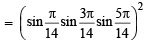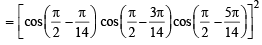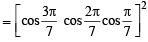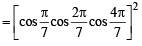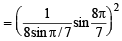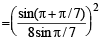=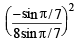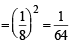Q.6. If K = sin(π/18) sin(5π/18) sin(7π/18), then the numerical value of K is _________ . (1993 - 2 Marks)
Ans.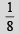Sol.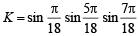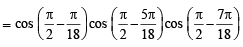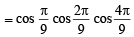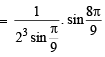[Using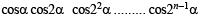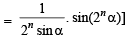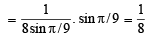Q.7. If A > 0,B>0 and A + B = π/3, then the maximum value of tan A tan B is_________ . (1993 - 2 Marks)
Ans.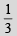Sol. A + B = π/3 ⇒ tan (A +B ) =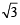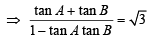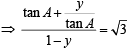[where y = tan A tan B]

⇒ tan2 A +(y – 1) tan A + y = 0

For real value of tan A, 3(y – 1)2 – 4y ≥ 0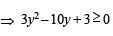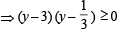⇒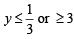But A, B > 0 and A + B = π/3
⇒ A, B  < π/3

⇒ tan A tan B < 3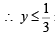i.e.,  max. value of y is 1/3.

Q.8. General value of θ satisfying the equation tan2θ + sec 2θ = 1 is_________ . (1996 - 1 Mark)
Ans.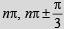Sol. tan2θ + sec 2θ= 1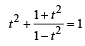where t = tanθ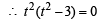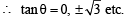which means θ = nπ and θ =nπ±π/3

Q.9. The real roots of the equation cosx + sin4 x=1 in the interval (–π, π) are ______, ______, and _________ . (1997 - 2 Marks)
Ans.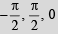Sol. cos7 x  = 1– sin4 x = (1– sin2 x) (1 + sin2 x) = cos2 x (1 + sin2 x)
∴ cos x = 0
or x = π/2, – π/2
or  cos5 x = 1 + sin2 x   or  cos5 x – sin2 x = 1
Now maximum value of each cos x or  sin x is 1.
Hence the above equation will hold  when cos x = 1 and sin x = 0. Both these imply x = 0
Hence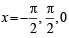Offer running on EduRev: Apply code STAYHOME200 to get INR 200 off on our premium plan EduRev Infinity!

## Maths 35 Years JEE Main & Advanced Past year Papers

111 docs|70 tests

,

,

,

,

,

,

,

,

,

,

,

,

,

,

,

,

,

,

,

,

,

,

,

,

,

,

,

,

,

,

;